Discretization method

splitting method

A grid method for solving non-stationary problems with one or more spatial variables, in which the passage from a given time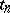to the next time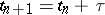is realized at the expense of a sequential solution of the grid analogues of related non-stationary problems with a smaller number of spatial variables (see ). One can often find methods in this class such that: 1) the whole passage from a grid layer at a moment of timeto a new grid layer at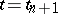is simple enough to be realized using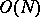arithmetical operations, whereis the number of nodes in the spatial grid; 2) absolute stability of the method is guaranteed; and 3) an acceptable precision of the method is guaranteed (existence of some form of approximation). Discretization methods are used fairly extensively in the practical solution of higher-dimensional problems of mathematical physics, such as those involving linear and non-linear systems of parabolic, hyperbolic or mixed type (see ).

For a problem withspatial variables, the passage fromto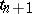by a discretization method can usually be carried out usingauxiliary (fractional) steps:(1)

whereis the matrix corresponding to the difference approximation of a certain differential operator, containing only derivatives with respect to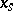(a one-dimensional differential operator), and the right-hand side of (1) can be easily calculated. By a corresponding enumeration of the unknowns, connected with the choice of the direction, the matricesusually become diagonal and the solution of (1) for everyreduces to the multiple solution of one-dimensional difference systems in the direction. Hence a discretization method is often called either an alternating-directing method (see also Variable-directions method) or a method of fractional steps (cf. Fractional steps, method of).

As a typical example, consider the equation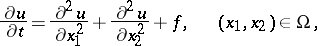with initial conditionand boundary condition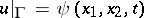, where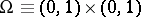andis the boundary of. The following method can be used on a square grid with step: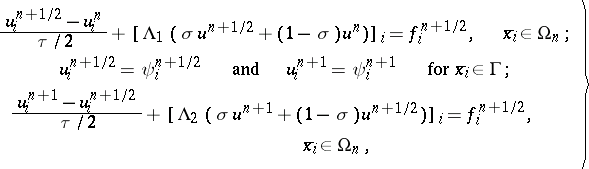where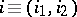,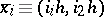,,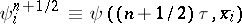,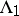and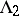are the simplest difference approximations to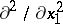and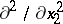,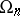is the set of all interior nodes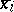, and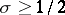.

There are two alternative approaches to the theory of discretization methods. In one of them the intermediate steps do not differ essentially from integral steps, and the difference equations in fractional steps and the boundary conditions for them, being similar to those in method , are carried out in the same way. One can thus expect thatand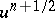will serve as approximations to the solution of the original problem at the moments of timeand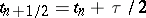. This approach is based on the use of the notions of a splitting scheme and a total approximation (see ). Schemes of such a type are often called locally one-dimensional schemes or additive schemes. They can also be treated as ordinary difference schemes for a certain equation with strongly-oscillating coefficients, whose solution must be close to that of the original problem (see ). The merits of this approach are in its simplicity and generality. For example, generalizations of method

also exist in the case of curvilinear domainsand for more general problems. The level of precision of the methods obtained when following this course is usually not very high. There are well-known and sometimes successfully applied variants of discretization methods in which the splitting takes place with respect to the physical processes rather than the spatial variables (see , ).

A second approach to the plan of analysis of stability and convergence dispenses with the need to consider fractional steps. Both the difference scheme itself and the approximation are treated in the traditional way. The unusualness of the difference scheme is to be found only at a higher level of the scheme, where there appears an unusual difference operator. For example, instead of method

one considers the method(3)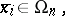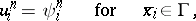where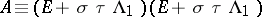andis the identity operator. Such operatorsare usually called split or factorized operators. The fractional steps are only connected with the method for solving the resulting system, and for one and the same scheme (3) one can introduce various methods, with boundary conditions chosen accordingly. Schemes of type (3) can be treated as ordinary weighted schemes for an-equation such aswhere the solutions differ from the solution of the original problem by(see ). In the case of a domainmade up of rectangles, the matrices of the systems arising in methods of type (3) cannot be written as a product of "one-dimensional" matrices. Nevertheless, solutions of similar systems can be found usingarithmetical operations (see ), and operators of this type are called generalized split operators. In investigating the stability and convergence of schemes with split and generalized split operators, a major role is played by the method of energy inequalities (see , , ).